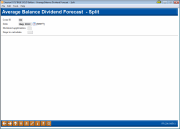# Dividend/Interest Split Rate Forecasting (1)

 Screen ID:  Screen Title:  Panel Number:IFEABD-01 Average Balance Dividend Forecast - Split 201Click here to magnify

Access this screen by selecting Tool #344: Div/Int Split Rate Forecasting

The Dividend/Interest Split Rate Forecasting tool is used to perform “what if” analysis, using actual member data. Like the Base Rate Forecasting tool, this tool shows the results of changes to dividend rates. However, this tool also lets you group accounts based on average minimum balance. This could be used to forecast split rates, or simply to see the status of your current accounts. No actual changes to member accounts are made; the tool simply lets you forecast what would happen if rates were to be changed.

This analysis is designed to look at a single product and the effect of split rates. In addition, this split analysis assumes the non-plateau rate calculation

• This tool can analyze how dividends/interest were paid on a previous month, with accurate numbers by tier so that this tool can be used for forecasting future rate changes.

• Data for previous month forecasting will be available with June 2008 end of month data.

This model also looks at the summation of the average balance of each member. The difference is that the member’s average balance will land the account on the effective rate tier based on the average. This model also counts the number of accounts affected by each tier and rate, which provides valuable information when considering rate changes.

## What the Forecasting Tool Can Do for Your Credit Union

• Display a clear and effective model of how a single product can approach different groups of accounts and members through split rate pricing.

• Measure and display these different groups for quantitative analysis based on “what if” scenarios.

• Stress the quantitative difference between the dollar effect of a rate change vs. the percentage of members affected.

## Setting Up the Forecast

This first screen is used to enter the variables used when performing the forecast calculation. Complete all fields (described below) and use Enter to proceed.

The system will generate the database for calculation of the forecast, and then display the initial results on the second screen. This may take a few minutes depending on the number of accounts measured, the activity level of the computer, and the number of products being measured. Once the model has been built, keep in mind that changes can be made without having to return and reset these initial parameters here.

• CU*TIP: The screen will remember your balance ranges and other settings for the next time you use the tool.

## Field Descriptions

 Field Name Description Corp ID Enter the corporation ID for the products to be included in the forecast. Month/year This dictates whether the system should use the credit union’s current trial balance position (including the time and date when the model is calculated), or whether the system should measure using the end-of-day, end-of-month files generated at the end of a previous month. For accounting examination, the key measurement periods during the year are the 12 end-of-day, end-of-month dates. However, the day-to-day manager may find some interesting keys to understanding the credit union’s money positions by varying the times and the days measured throughout a monthly period. Remember, however, that the only verifiable and consistently reported dates are end-of-day, end-of-month. Dividend application Enter the dividend application of the product for which the forecast is to be calculated. This field is required in order for the forecast to be generated. Days to calculate Use this field to indicate the number of days for which dividend expense should be shown. For example, if you want to see a month’s worth of dividends, enter 30 here. After the model has been generated, this figure may be changed on the next screen and the forecast recalculated.